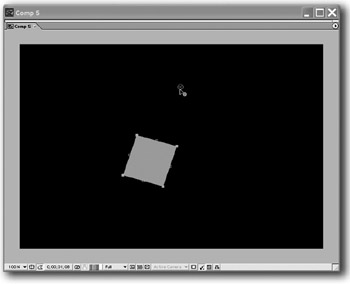# Useful Expressions II

Pendulum By Dan Ebberts

This is an expression you can use to generate a decaying pendulum motion.

The pendulum consists of a rectangular solid and a square solid with a circular mask applied. The anchor point of the rectangular solid has been moved to the upper edge. The square solid is parented to the retangular solid.

Apply the following expression to the rotation property of the rectangular solid:

` freq = 1.0; //oscillations per second amplitude = 50; decay = 0.7; amplitude*Math.sin(freq*time*2*Math.PI)/Math.exp(decay*time) `After Effects On the Spot[c] Time-Saving Tips and Shortcuts from the Pros
ISBN: 1578202396
EAN: N/A
Year: 2006
Pages: 447